Your browser (Internet Explorer 8 or lower) is out of date. It has known security flaws and may not display all features of this and other websites. Learn how to update your browser.

X

# Predator-Prey Interactions (SD)

This tutorial describes how to construct a model of the interactions between a predator species (wolves) and a prey species (moose). Before starting the tutorial, make sure you have familiarized yourself with how to create primitives and run models.
First, let's create the structure to model a small population of moose.
1. Create a new Stock named Moose.
2. Create a new Flow going from empty space to the primitive Moose. Name that flow Moose Births.
3. Create a new Flow going from the primitive Moose to empty space. Name that flow Moose Deaths.
4. The model diagram should now look something like this: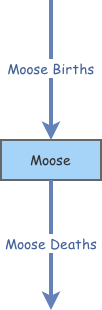Now that the structure has been defined, let's enter the equations to define how our moose population behaves. We'll assume 150 moose to start and constant birth- and death-rates.
5. Change the Initial Value property of the primitive Moose to 150.
6. Change the Flow Rate property of the primitive Moose Births to 0.16*[Moose].
7. Change the Flow Rate property of the primitive Moose Deaths to 0.10*[Moose].
8. Run the model. Here are sample results: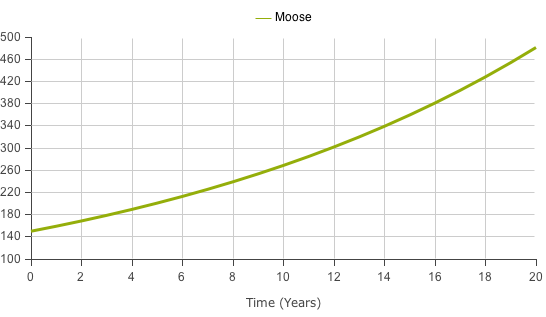We see an exponential growth pattern to this model. That because the birth-rate is higher than the death-rate. If the reverse had been true, we would have seen a population decline over time.

Now let's create a population of wolves.
9. Create a new Stock named Wolves.
10. Create a new Flow going from empty space to the primitive Wolves. Name that flow Wolf Births.
11. Create a new Flow going from the primitive Wolves to empty space. Name that flow Wolf Deaths.
12. The model diagram should now look something like this: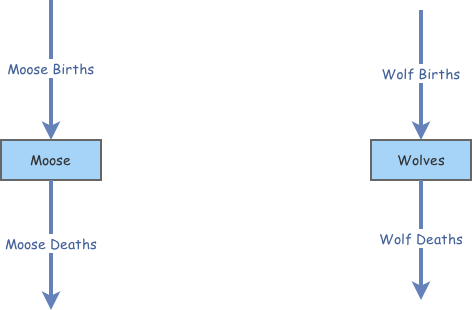Now we can enter equations to define the behavior of the wolf population.
13. Change the Initial Value property of the primitive Wolves to 100.
14. Change the Flow Rate property of the primitive Wolf Births to 0.2*[Wolves].
15. Change the Flow Rate property of the primitive Wolf Deaths to 0.12*[Wolves].
16. Run the model. Here are sample results: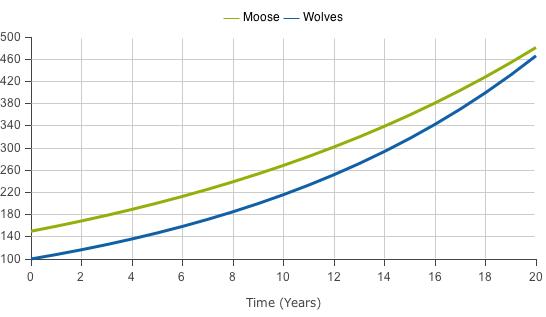So far, so good.

Before we have the two populations interact, let's make our model more modular by putting all the rate constants into separate variable primitives. This will make the model easier to mantain.
17. Create a new Variable named Moose Birth Rate.
18. Create a new Variable named Wolf Birth Rate.
19. Create a new Variable named Moose Death Rate.
20. Create a new Variable named Wolf Death Rate.
21. The model diagram should now look something like this: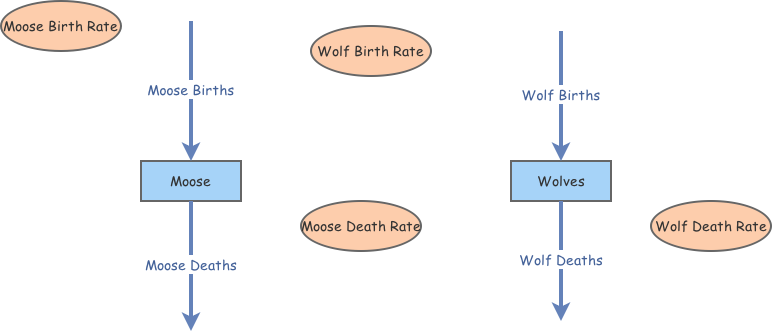22. Create a new Link going from the primitive Moose Birth Rate to the primitive Moose Births.
23. Create a new Link going from the primitive Wolf Birth Rate to the primitive Wolf Births.
24. Create a new Link going from the primitive Moose Death Rate to the primitive Moose Deaths.
25. Create a new Link going from the primitive Wolf Death Rate to the primitive Wolf Deaths.
26. The model diagram should now look something like this: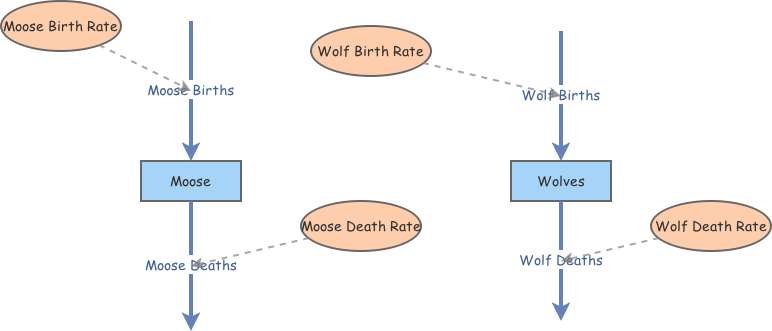Let's add the equations for the new cariables to complete this modularization.
27. Change the Flow Rate property of the primitive Moose Births to [Moose Birth Rate]*[Moose].
28. Change the Equation property of the primitive Moose Birth Rate to 0.16.
29. Change the Flow Rate property of the primitive Moose Deaths to [Moose Death Rate]*[Moose].
30. Change the Equation property of the primitive Moose Death Rate to 0.10.
31. Change the Flow Rate property of the primitive Wolf Births to [Wolf Birth Rate]*[Wolves].
32. Change the Equation property of the primitive Wolf Birth Rate to 0.2.
33. Change the Flow Rate property of the primitive Wolf Deaths to [Wolf Death Rate]*[Wolves].
34. Change the Equation property of the primitive Wolf Death Rate to 0.12.
That's it! Let's check to make sure we get the same results as before.
35. Run the model. Here are sample results: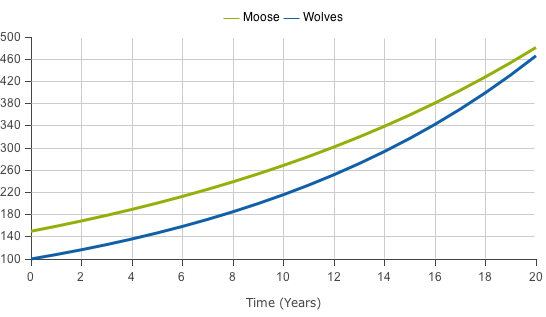Now it's time to make the wolf and moose populations interact. In this model extension, wolves will chase and consume the moose. The more moose they catch, the faster the wolves reproduce, and the faster the moose population declines. We'll make the moose death-rate dependent on the number of wolves, and the wolf birth-rate dependent on the number of moose.
36. Create a new Link going from the primitive Moose to the primitive Wolf Birth Rate.
37. Create a new Link going from the primitive Wolves to the primitive Moose Death Rate.
38. The model diagram should now look something like this: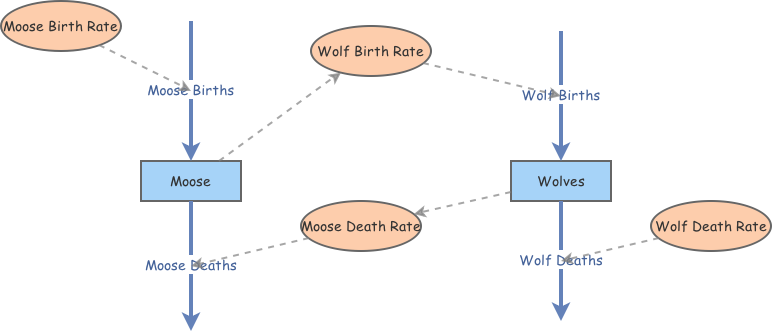39. Change the Equation property of the primitive Wolf Birth Rate to 0.001*[Moose].
40. Change the Equation property of the primitive Moose Death Rate to 0.0008*[Wolves].
41. Run the model. Here are sample results: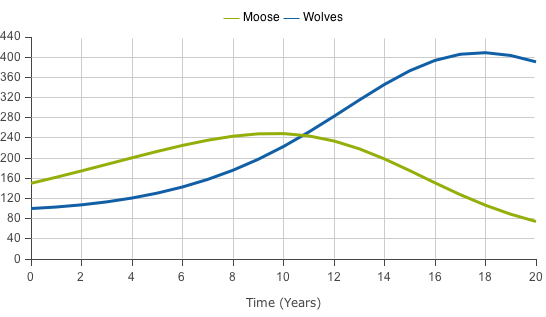That's getting interesting! Let's increase the length and accuracy of our simulation.
42. Change the Analysis Algorithm property of the Time Settings to RK4.
43. Change the Simulation Time Step property of the Time Settings to 0.5.
44. Change the Simulation Length property of the Time Settings to 100.
45. Run the model. Here are sample results: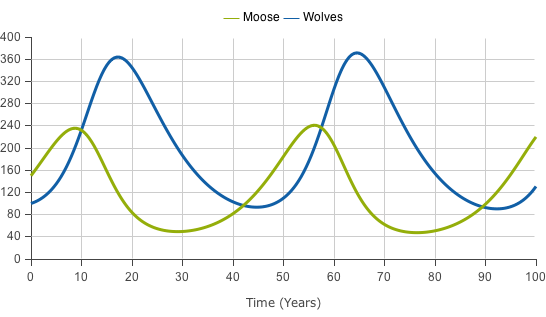Great! The oscillatory behavior we see is typical of some predator-prey systems. The wolves kill many of the moose, but then the wolves have nothing to eat. This leads the wolf population to drop, allowing the moose population to recovery. This repeats in an ongoing cycle.

We can also configure the display to use a scatter-plot instead of a time-series to show the phase-plane oscillatory behavior clearly.
46. Run the model. Here are sample results: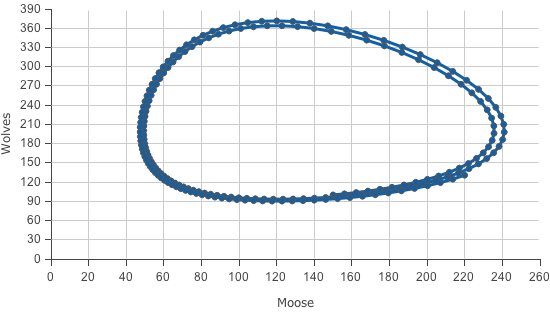There are many parameters in this model you can experiment with. This form of model what is known as the Lotka-Volterra Model in the modeling community.